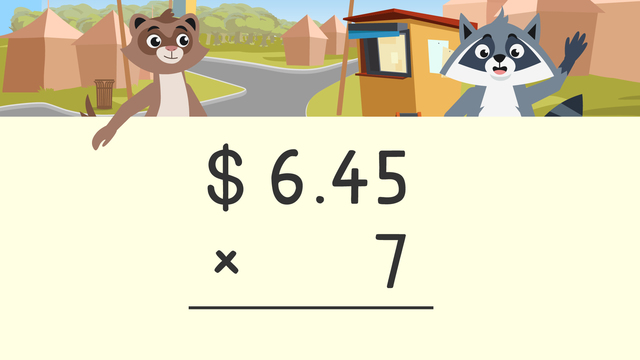# Multiplication and MoneyRate this video

Be the first to rate this video!

The authorTeam Digital
Multiplication and Money
CCSS.MATH.CONTENT.4.MD.A.2

## Information about the videoMultiplication and Money

### What is Money Multiplication?

Zuri and Freddie want to visit the Bee’s Knees Adventure park, but the tickets are too expensive! They decide to have a bake sale to earn money for the tickets. However, Zuri and Freddie are having trouble keeping track of the money! Follow along as they learn more about how to multiply money and multi-digit multiplication. Below we will explore more about money and multiplying with decimal points.

#### How to do Multiplication with Money

How do you multiply with money? Many people find the total cost of something by ADDING up money. But, you can use multiplication to count money in a more efficient way. You can multiply the cost of one item by how many people buy it. When you multiply money with decimal points it can be helpful to take out the decimal points while multiplying as whole numbers and then put them back in at the end. When multiplying money with decimal points…

• Start by setting up the equation.

• Next, multiply as whole numbers.

• After that, circle the digits to the right of the decimal point.

• Then, count the digits to the right of the decimal point.

• Finally, put the dollar sign on.

#### Multiplication Examples with Money

Zuri and Freddie are selling breakfast muffins to their neighbors. If one box costs \$4.25 and five people buy a box of muffins, how much money will Zuri and Freddie have made?Start by creating a multiplication equation, here it is four dollars and twenty-five cents times five.

Next, multiply as whole numbers. four hundred twenty five times five equals two thousand one hundred twenty-five.

Then, circle the digits to the right of the decimal point and put the decimal point back in.

Finally, put the dollar sign on to find the cost!

Zuri and Freddie will receive twenty-one dollars and twenty-five cents from selling their breakfast muffins.

#### Multiplication with Money – Summary

Remember with multiplication of money ...

• Start by setting up the equation.

• Next, multiply as whole numbers.

• After that, circle the digits to the right of the decimal point.

• Then, count the digits to the right of the decimal point.

• Finally, put the dollar sign on.

#### Money Multiplication – Additional Practice

At the end of the video, there are exercises for continued practice of multiplying whole numbers by decimals and worksheets.